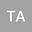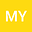••The Dirichlet and Neumann boundary value problems (BVPs) for the linear second-order scalar elliptic differential equation with variable coefficients in a bounded two-dimensional domain are considered. The PDE right-hand side belongs to $H^{-1}(\Omega)$ or $\widetilde{H}^{-1}(\Omega),$ when neither classical nor canonical conormal derivatives of solutions are well defined. Using the two-operator approach and appropriate parametrix (Levi function) each problem is reduced to two different systems of two-operator boundary-domain integral equations (BDIEs). Although the theory of BDIEs in 3D is well developed, the BDIEs in 2D need a special consideration due to their different equivalence properties. As a result, we need to set conditions on the domain or on the associated Sobolev spaces to ensure the invertibility of corresponding parametrix-based integral layer potentials and hence the unique solvability of BDIEs. The equivalence of the two-operator BDIE systems to the original problems, BDIE system solubility, solution uniquness/nonuniquness and invertibility BDIE system are analyzed in the appropriate Sobolev (Bessel potential) spaces. It is shown that the BDIE operators for the Neumann BVP are not invertible, and appropriate finite-dimensional perturbations are constructed leading to invertibility of the perturbed operators.